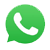Calculation Power Calculator Converting kVA to kW Converting kW to kVA Converting kW to HP Amperes when kVA is known kVA Required to run motors
 Guide to Standard Uints Kilo Volt Amperes kVA KiloWatts (1000 watts = 1 kW) kW Ampere (Volt-Amperes or Current) I Volts E Power Factor PE Percent Efficiency %EFF Horse Power HP

Power Requirement Calculator
 Phase Volts Required V Amperes I Power Factor = Power kW 1 3 .8 1.0

Converting kW to kVA
 kW = kVA

Converting kVA to kW
 kVA = kW

Converting kW to HP
 kW = HP

What size genset is needed to start a 3 phase electric motor Direct on Line (DOL) start
 HP of Motor = Generator kVA Required

Calculating Amperes (when you know kVA)
 Phase 1,2,3 Generator kVA Volts Required = Ampere I